## 5.259. min_size_set_of_consecutive_var

Origin

N. Beldiceanu

Constraint

$\mathrm{𝚖𝚒𝚗}_\mathrm{𝚜𝚒𝚣𝚎}_\mathrm{𝚜𝚎𝚝}_\mathrm{𝚘𝚏}_\mathrm{𝚌𝚘𝚗𝚜𝚎𝚌𝚞𝚝𝚒𝚟𝚎}_\mathrm{𝚟𝚊𝚛}\left(\mathrm{𝙼𝙸𝙽},\mathrm{𝚅𝙰𝚁𝙸𝙰𝙱𝙻𝙴𝚂}\right)$

Arguments
 $\mathrm{𝙼𝙸𝙽}$ $\mathrm{𝚍𝚟𝚊𝚛}$ $\mathrm{𝚅𝙰𝚁𝙸𝙰𝙱𝙻𝙴𝚂}$ $\mathrm{𝚌𝚘𝚕𝚕𝚎𝚌𝚝𝚒𝚘𝚗}\left(\mathrm{𝚟𝚊𝚛}-\mathrm{𝚍𝚟𝚊𝚛}\right)$
Restrictions
 $\mathrm{𝙼𝙸𝙽}\ge 1$ $\mathrm{𝙼𝙸𝙽}\le |\mathrm{𝚅𝙰𝚁𝙸𝙰𝙱𝙻𝙴𝚂}|$ $\mathrm{𝚛𝚎𝚚𝚞𝚒𝚛𝚎𝚍}$$\left(\mathrm{𝚅𝙰𝚁𝙸𝙰𝙱𝙻𝙴𝚂},\mathrm{𝚟𝚊𝚛}\right)$
Purpose

$\mathrm{𝙼𝙸𝙽}$ is the size of the smallest set of variables of the collection $\mathrm{𝚅𝙰𝚁𝙸𝙰𝙱𝙻𝙴𝚂}$ that all take their value in a set of consecutive values.

Example
 $\left(4,〈3,1,3,7,4,1,2,8,7,6〉\right)$ $\left(4,〈3,1,3,2〉\right)$

In the first example, the two parts $3,1,3,4,1,2$ and $7,8,7,6$ take respectively their values in the two following sets of consecutive values $\left\{1,2,3,4\right\}$ and $\left\{6,7,8\right\}$. Consequently, the corresponding $\mathrm{𝚖𝚒𝚗}_\mathrm{𝚜𝚒𝚣𝚎}_\mathrm{𝚜𝚎𝚝}_\mathrm{𝚘𝚏}_\mathrm{𝚌𝚘𝚗𝚜𝚎𝚌𝚞𝚝𝚒𝚟𝚎}_\mathrm{𝚟𝚊𝚛}$ constraint holds since the cardinality of the smallest set of variables is 4.

Typical
 $\mathrm{𝙼𝙸𝙽}>1$ $\mathrm{𝙼𝙸𝙽}<|\mathrm{𝚅𝙰𝚁𝙸𝙰𝙱𝙻𝙴𝚂}|$ $|\mathrm{𝚅𝙰𝚁𝙸𝙰𝙱𝙻𝙴𝚂}|>0$ $\mathrm{𝚛𝚊𝚗𝚐𝚎}$$\left(\mathrm{𝚅𝙰𝚁𝙸𝙰𝙱𝙻𝙴𝚂}.\mathrm{𝚟𝚊𝚛}\right)>1$
Symmetries
• Items of $\mathrm{𝚅𝙰𝚁𝙸𝙰𝙱𝙻𝙴𝚂}$ are permutable.

• All occurrences of two distinct values of $\mathrm{𝚅𝙰𝚁𝙸𝙰𝙱𝙻𝙴𝚂}.\mathrm{𝚟𝚊𝚛}$ can be swapped.

• One and the same constant can be added to the $\mathrm{𝚟𝚊𝚛}$ attribute of all items of $\mathrm{𝚅𝙰𝚁𝙸𝙰𝙱𝙻𝙴𝚂}$.

Arg. properties

Functional dependency: $\mathrm{𝙼𝙸𝙽}$ determined by $\mathrm{𝚅𝙰𝚁𝙸𝙰𝙱𝙻𝙴𝚂}$.

Counting
 Length ($n$) 2 3 4 5 6 7 8 Solutions 9 64 625 7776 117649 2097152 43046721

Number of solutions for $\mathrm{𝚖𝚒𝚗}_\mathrm{𝚜𝚒𝚣𝚎}_\mathrm{𝚜𝚎𝚝}_\mathrm{𝚘𝚏}_\mathrm{𝚌𝚘𝚗𝚜𝚎𝚌𝚞𝚝𝚒𝚟𝚎}_\mathrm{𝚟𝚊𝚛}$: domains $0..n$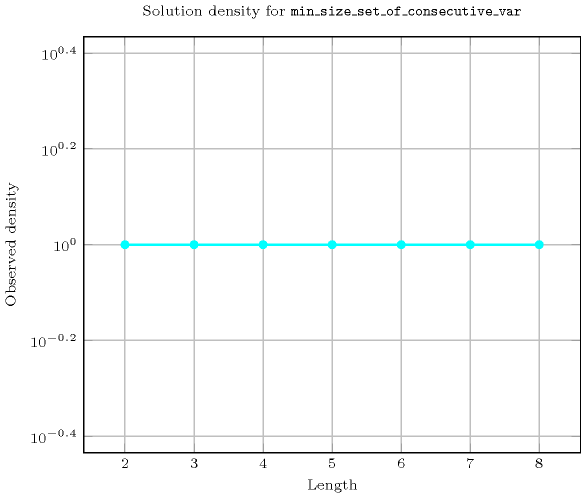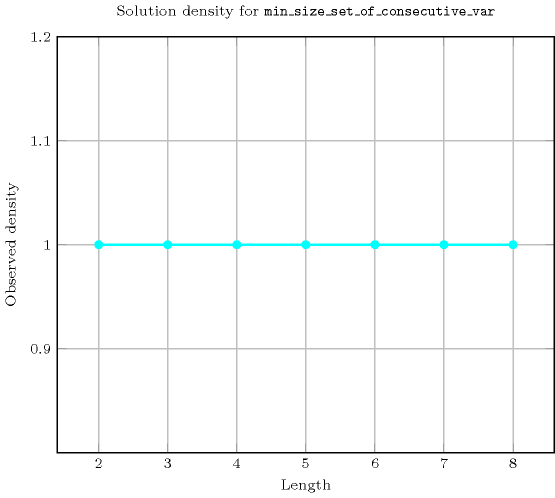Length ($n$)2345678
Total9646257776117649209715243046721
 Parameter value

1230276358057000106583422894984
27-13224803099052252211080412
3-34--135003324304590208
4--217---2293480
5---1716---
6----16159--
7-----176366-
8------2187637

Solution count for $\mathrm{𝚖𝚒𝚗}_\mathrm{𝚜𝚒𝚣𝚎}_\mathrm{𝚜𝚎𝚝}_\mathrm{𝚘𝚏}_\mathrm{𝚌𝚘𝚗𝚜𝚎𝚌𝚞𝚝𝚒𝚟𝚎}_\mathrm{𝚟𝚊𝚛}$: domains $0..n$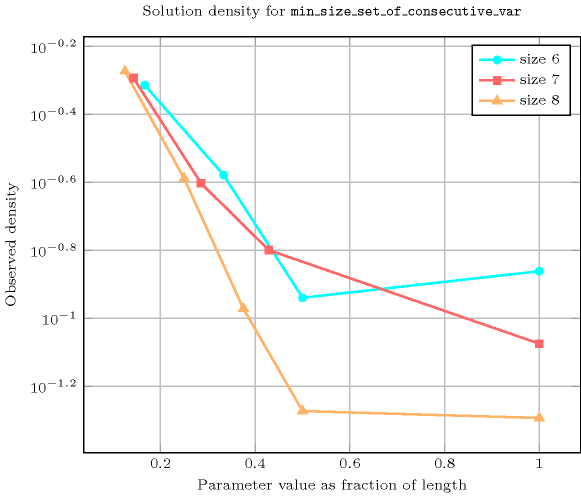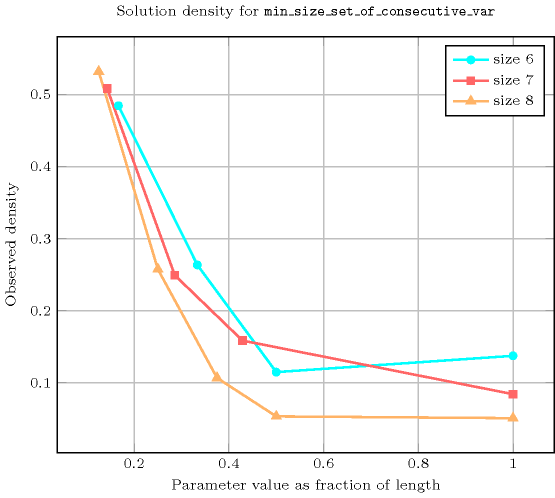Keywords
Arc input(s)

$\mathrm{𝚅𝙰𝚁𝙸𝙰𝙱𝙻𝙴𝚂}$

Arc generator
$\mathrm{𝐶𝐿𝐼𝑄𝑈𝐸}$$↦\mathrm{𝚌𝚘𝚕𝚕𝚎𝚌𝚝𝚒𝚘𝚗}\left(\mathrm{𝚟𝚊𝚛𝚒𝚊𝚋𝚕𝚎𝚜}\mathtt{1},\mathrm{𝚟𝚊𝚛𝚒𝚊𝚋𝚕𝚎𝚜}\mathtt{2}\right)$

Arc arity
Arc constraint(s)
$\mathrm{𝚊𝚋𝚜}\left(\mathrm{𝚟𝚊𝚛𝚒𝚊𝚋𝚕𝚎𝚜}\mathtt{1}.\mathrm{𝚟𝚊𝚛}-\mathrm{𝚟𝚊𝚛𝚒𝚊𝚋𝚕𝚎𝚜}\mathtt{2}.\mathrm{𝚟𝚊𝚛}\right)\le 1$
Graph property(ies)
$\mathrm{𝐌𝐈𝐍}_\mathrm{𝐍𝐒𝐂𝐂}$$=\mathrm{𝙼𝙸𝙽}$

Graph model

Since the arc constraint is symmetric each strongly connected component of the final graph corresponds exactly to one connected component of the final graph.

Parts (A) and (B) of Figure 5.259.1 respectively show the initial and final graph associated with the first example of the Example slot. Since we use the $\mathrm{𝐌𝐈𝐍}_\mathrm{𝐍𝐒𝐂𝐂}$ graph property, we show the smallest strongly connected component of the final graph.

##### Figure 5.259.1. Initial and final graph of the $\mathrm{𝚖𝚒𝚗}_\mathrm{𝚜𝚒𝚣𝚎}_\mathrm{𝚜𝚎𝚝}_\mathrm{𝚘𝚏}_\mathrm{𝚌𝚘𝚗𝚜𝚎𝚌𝚞𝚝𝚒𝚟𝚎}_\mathrm{𝚟𝚊𝚛}$ constraint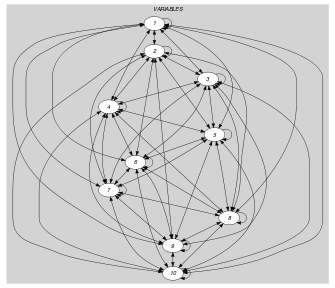(a)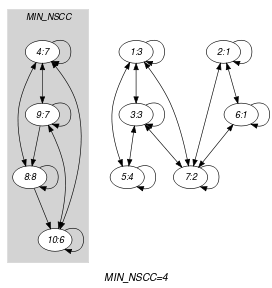(b)